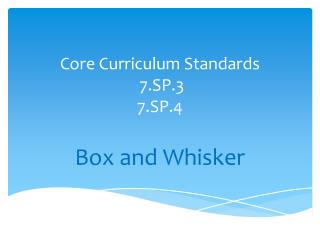# Core Curriculum Standards 7.SP.3 7.SP.4 - PowerPoint PPT PresentationDownload PresentationCore Curriculum Standards 7.SP.3 7.SP.4

Presentation Description
Download Presentation## Core Curriculum Standards 7.SP.3 7.SP.4

- - - - - - - - - - - - - - - - - - - - - - - - - - - E N D - - - - - - - - - - - - - - - - - - - - - - - - - - -
##### Presentation Transcript

1. Core Curriculum Standards 7.SP.37.SP.4 Box and Whisker

2. Definition of Box and Whisker A box-and-whisker plot shows the distribution of a set of data along a number line, dividing the data into four parts using the median and quartiles.

3. How to create a box and whisker First: To create a box-and-whisker plot, you start by ordering your data (putting the values in numerical order), if they aren't ordered already. Then you find the medianof your data. The median divides the data into two halves. http://www.wikihow.com/Find-the-Median-of-a-Set-of-Numbers

4. Second: You have three points: the first middle point (the median), and the middle points of the two halves (what I call the "sub-medians"). These three points divide the entire data set into quarters, called "quartiles". The top point of each quartile has a name, being a "Q" followed by the number of the quarter. So the top point of the first quarter of the data points is "Q1", and so forth. Note that Q1 is also the middle number for the first half of the list, Q2 is also the middle number for the whole list, Q3 is the middle number for the second half of the list, and Q4 is the largest value in the list. Once you have these three points, Q1, Q2, and Q3, you have all you need in order to draw a simple box-and-whisker plot. Here's an example of how it works.